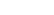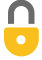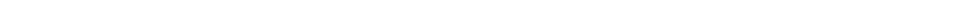Problem 15
Q

# At room temperature, an oxygen molecule, with mass of $5.31 \times 10^{-26} \textrm{ kg}$, typically has a kinetic energy of about $6.21 \times 10^{-21} \textrm{ J}$. How fast is it moving?A
$484 \textrm{ m/s}$In order to watch this solution you need to have a subscription.VIDEO TRANSCRIPT

This is Giancoli Answers with Mr. Dychko. Kinetic energy is one-half mass times velocity squared and we can solve for v by multiplying both sides by 2 over m and then we take the square root of both sides and we get v. So v is the square root of 2 times kinetic energy divided by mass so that's square root of 2 times the kinetic energy of 6.21 times 10 to the minus 21 joules, for an oxygen molecule, divided by an oxygen molecule's mass of 5.31 times 10 to the negative 26 kilograms that gives us speed of about 484 meters per second.

Giancoli Answers, including solutions and videos, is copyright © 2009-2023 Shaun Dychko, Vancouver, BC, Canada. Giancoli Answers is not affiliated with the textbook publisher. Book covers, titles, and author names appear for reference purposes only and are the property of their respective owners. Giancoli Answers is your best source for the 7th and 6th edition Giancoli physics solutions.